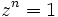# Tour:Mind's eye test one (beginners)

PREVIOUS: Entertainment menu one (beginners)| UP: Introduction one | NEXT: Examples peek one (beginners)
NEXT SECTION MIND'S EYE TEST: Mind's eye test two
General instructions for the tour | Pedagogical notes for the tour | Pedagogical notes for this part

It's time to do a quick mind's eye test of what we've seen so far. Look at the questions below, and try to hold the answers to these questions in your mind's eye. If you find yourself faltering, write down, or revisit the relevant pages of the tour:

## Definitions and conditions

1. Define an abelian group from scratch (without assuming the definition of group; so your definition subsumes the definition of group)
2. Hold in the mind the set of conditions that a subset of a group must satisfy to be a subgroup.
3. Hold in the mind the procedure to check that a set with a binary operation, gives rise to a group.

## Proofs

1. Prove that every group is nonempty, and prove that every group contains a one-element subgroup that looks like the trivial group.
2. Prove that the trivial group is abelian.
3. Prove that any subgroup of an abelian group is abelian.
4. Suppose$G$ is a group,$K$ is a subgroup of$G$, and$H$ is a subgroup of$K$. Prove that$H$ is a subgroup of$G$.

## Example verification

These examples are just for confirming that you can verify the conditions for a group. More examples are available in the examples peek page, where the examples are developed a bit more.

1. Prove that the set of positive integers, under addition, do not form a group.
2. Prove that the set of all integers, under multiplication, do not form a group.
3. Prove that the set of positive reals, under multiplication, form an abelian group.
4. Let$a$ be any real number. Prove that the real numbers form an abelian group under the following operation$*$:$x * y := x + y + a$. Find the identity element and inverse map.
5. Prove that for a finite set$S$, the set of all bijective maps from$S$ to$S$, forms a group under composition. This group is termed the symmetric group on$S$.
6. NEEDS SOME THOUGHT: Let$G$ be the set of all complex numbers$z$ for which there exists a positive integer$n$ such that$z^n = 1$. Prove that$G$ is a group under multiplication.